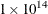The HPSUMMARY Procedure

WEIGHT Statement

Subsections:

• WEIGHT weight-variable;

The WEIGHT statement specifies weights for observations in the statistical calculations.

Required Argument

weight-variable

specifies a numeric weight-variable whose values weight the values of the analysis variables. The values of weight-variable do not have to be integers. Table 10.7 describes how PROC HPSUMMARY treats various values of weight-variable.

Table 10.7: Responses to Values of weight-variable

Value

PROC HPSUMMARY Response

0

Counts the observation in the total number of observations

Less than 0

Converts the value to zero and counts the observation in the total number of observations

Missing

Excludes the observation

To exclude observations that contain negative and zero weights from the analysis, use the EXCLNPWGT option in the PROC HPSUMMARY statement.

Caution: Single extreme weight values can cause inaccurate results. When one (and only one) weight value is many orders of magnitude larger than the other weight values (for example, 49 weight values of 1 and one weight value of), certain statistics might not be within acceptable accuracy limits. The affected statistics are based on the second moment (such as standard deviation, corrected sum of squares, variance, and standard error of the mean). Under certain circumstances, no warning is written to the SAS log.

To compute weighted quantiles, you must use QMETHOD= OS in the PROC statement. Skewness and kurtosis are not available with the WEIGHT statement.

PROC HPSUMMARY does not compute MODE when a weight variable is active. Instead, you can try using the UNIVARIATE procedure when MODE needs to be computed and a weight variable is active.

If you use the WEIGHT= option in a VAR statement to specify a weight variable, then PROC HPSUMMARY uses this variable instead to weight those VAR statement variables.

When you use the WEIGHT statement, consider which value of the VARDEF= option is appropriate. See the section Keywords and Formulas for more information.